## 线性表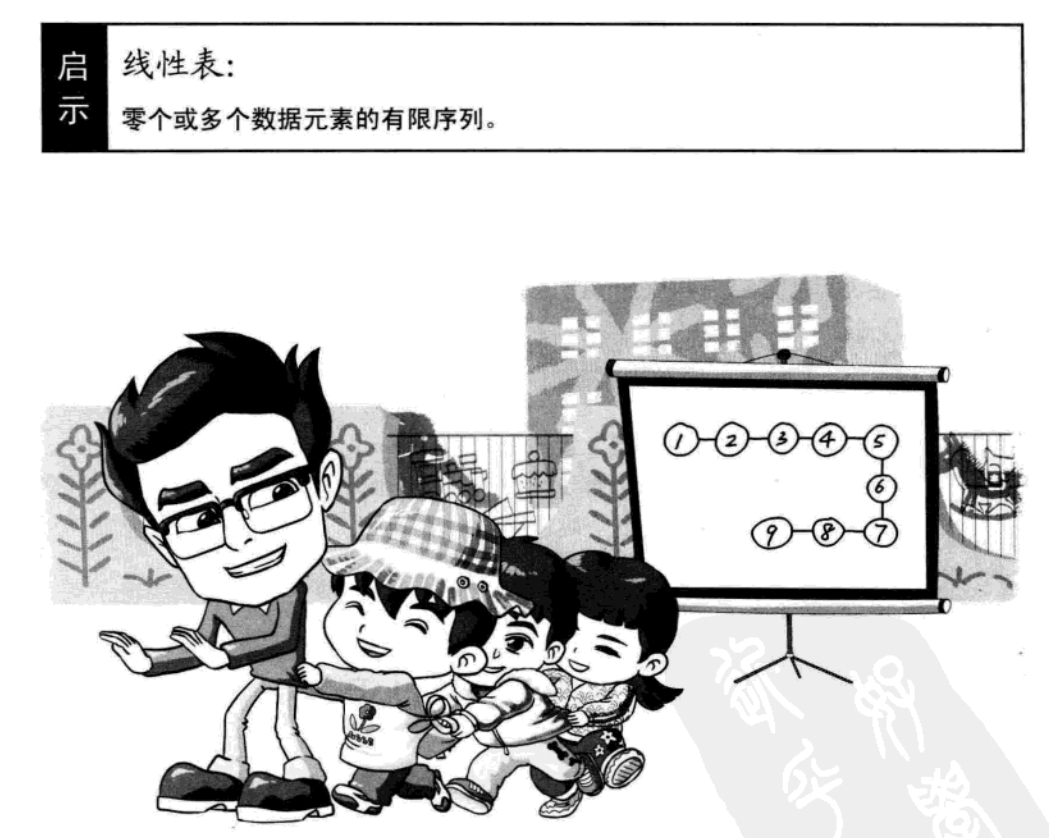• 数据有限

• 元素之间是有顺序

• 若元素存在多个

• 第一个元素无前驱
• 最后一个元素无后继
• 其他每个元素都有且只有一个前驱和后继

### 数学定义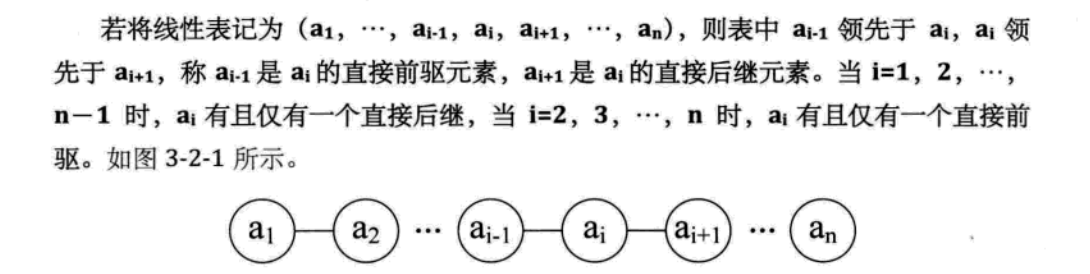### 抽象数据类型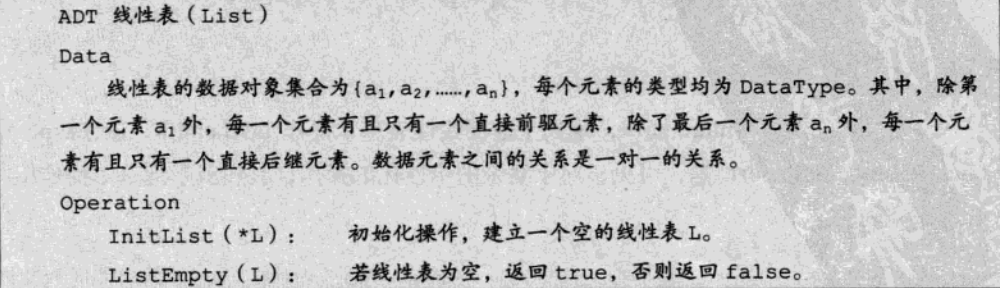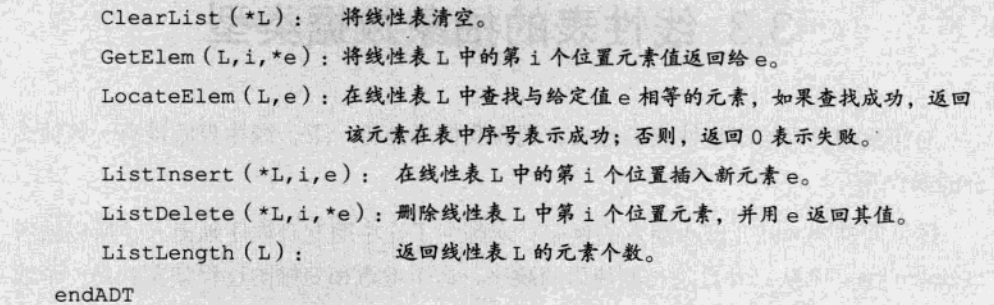## 线性表的顺序存储结构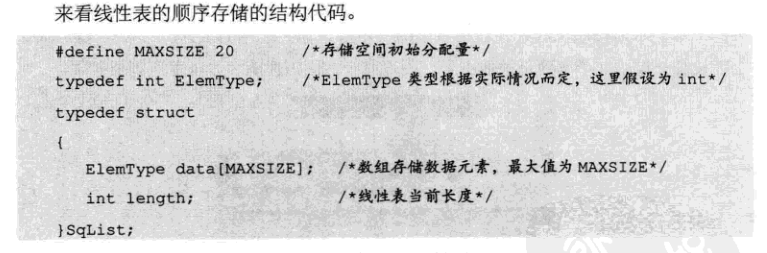• 存储空间 数组 data
• 最大容量 maxSize
• 当前长度 length

### 代码实现

``````package com.linearlist;

/**
* 线性表顺序存储
*/
public class LinearList {
private int maxSize;
private int length;
private int[] arr;

public LinearList(int maxSize) {
// 初始化传入一个最大容量
this.maxSize = maxSize;
this.arr = new int[maxSize];
this.length = 0;
}

public boolean isEmpty() {
// 空返回真
return arr == null || length == 0;
}

public boolean isFull() {
return length == maxSize;
}

// 获取某个元素
public int getElem(int i) {
if (isEmpty()) {
throw new RuntimeException("线性表为空");
}
return arr[i - 1];
}

public void insertElem(int i, int num) {
//在第i个位置插入元素num
if (isFull()) {
System.out.println("挤不下了~兄弟");
return;
}
if (i < 0 || i > maxSize) {
System.out.println("输入异常");
return;
}
// 从i位置后面的人都要后移，包括i
int j = ++length;
for (; j >= i; j--) {
arr[j] = arr[j - 1];
}
// 腾出位置后，即可插入
arr[j] = num;
}

public int deleteElem(int i) {
//在第i个位置插入元素num
if (isEmpty()) {
throw new RuntimeException("删完了");
} else if (i < 0 || i > length) {
throw new RuntimeException("输入异常");
}
int temp = arr[i - 1];

// 从删除的位置后一个向前移动
for (int j = i - 1; j < length - 1; j++) {
arr[j] = arr[j + 1];
}
length--;
// deleteElem(4)
// 1 2 3 4
// 0 1 2 3
// 4
return temp;
}

public void list() {
if (isEmpty()) {
System.out.println("无数据");
return;
}
for (int i =0;i<length;i++) {
System.out.print(arr[i] + "\t");
}
}
}
``````

### 优缺点

• 无须为表中的元素之间的逻辑关系而添加额外的存储空间
• 可以快速的存取表中的任一位置的元素

• 插入和删除需要移动大量元素
• 当线性表变化大时，难以确定存储空间的容量
• 造成存储空间的“碎片”

`数组本质`：物理上存储在一组联系的地址上，也就是数据结构中的顺序存储`物理结构`

`线性表本质`：线性表是数据结构中的`逻辑结构`

**线性表根据存储结构的不同可以分为 **

• 顺序表，通过数组实现（顺序存储结构）
• 链表，通过链式存储实现

## 线性表的链式存储结构

### 基本概念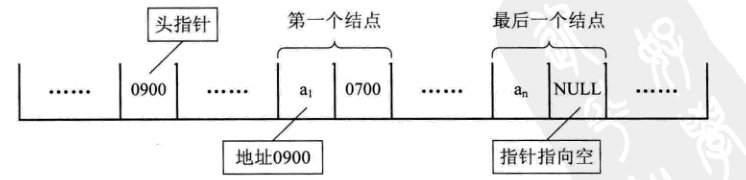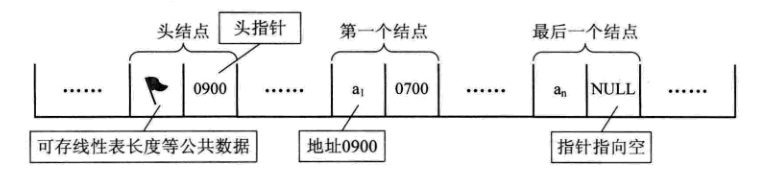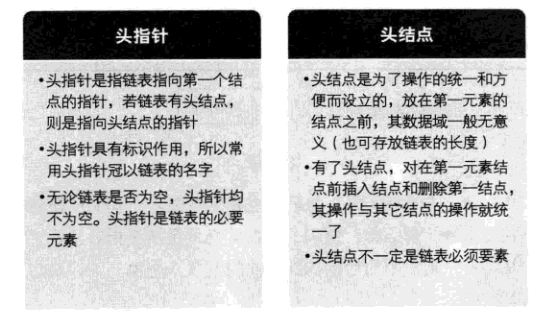### 单链表的实现

HerNode.java

``````/**
* 定义一个节点
*/
class HeroNode {
public int no;
public String name;
public String nickname;
public HeroNode next;

public HeroNode() {
}

public HeroNode(int no, String name, String nickname) {
this.no = no;
this.name = name;
this.nickname = nickname;
}

@Override
public String toString() {
return "HeroNode{" +
"no=" + no +
", name='" + name + '\'' +
", nickname='" + nickname + '\'' +
'}';
}
}
``````

SingleLinkedList.java

``````import HeroNode;

public class SingleLinkedList {
// 先初始化一个头结点，头结点是寻找链表开始，不要动它
private HeroNode head = new HeroNode(0, "", "");

public HeroNode getHead() {
return head;
}

public void setHead(HeroNode head) {
this.head = head;
}

/**
* 头插法添加新节点
*/
public void addHead(HeroNode heroNode) {
heroNode.next = head.next;
head.next = heroNode;
}

/**
* 尾插法添加新节点
* @param heroNode 新节点
*/
public void add(HeroNode heroNode) {
// 1.首先遍历到尾结点
HeroNode temp = head;
while (temp.next != null) {
temp = temp.next;
}
// 2.将尾结点的next指向新节点
temp.next = heroNode;
}

/**
* 根据排名插入数据
* @param heroNode 新节点
*/
public void addByOrder(HeroNode heroNode) {
// 1.首先找到新节点的插入位置
HeroNode temp = head;
// 如果找到尾结点还没有找到合适的位置，则尾插到最后
while (temp.next != null && temp.next.no <= heroNode.no) {
temp = temp.next;
if (temp.no == heroNode.no) {
System.out.println(heroNode.name+"已存在,不可添加");
return;
}
}
// 2.新节点指向后节点
heroNode.next = temp.next;
// 3.原节点指向新节点
temp.next = heroNode;
}

/**
* 根据编号更新节点数据
*/
public void update(HeroNode heroNode) {

// 1.首先找到新节点的插入位置
HeroNode temp = head;
while (temp.next != null && temp.no != heroNode.no) {
temp = temp.next;
}
// 如果跳出循环还没有匹配，就是找不到对应的节点
if (temp.no != heroNode.no) {
System.out.println("该编号不存在");
} else {
temp.name = heroNode.name;
temp.nickname = heroNode.nickname;
}
}

/**
* 删除节点
*/
public void delete(int no) {
if (head.next == null) {
System.out.println("双向链表为空");
return;
}
// 首先找到删除节点的【前一个】节点
HeroNode temp = head;
while (temp.next != null && temp.next.no != no) {
temp = temp.next;
}
// 如果没没有遍历到最后一个节点说明找到了匹配的节点
if (temp.next != null) {
// 跳过匹配节点的链接
temp.next = temp.next.next;
} else {
System.out.println(no + "节点不存在");
}
}
/**
* 打印链表信息
*/
public void list() {
if (head.next == null) {
System.out.println("链表为空");
return;
}
// 临时变量遍历
HeroNode temp = head;
while (temp.next != null) {
temp = temp.next;
System.out.println(temp.toString());
}
}
}
``````

## 单链表和顺序存储结构的优缺点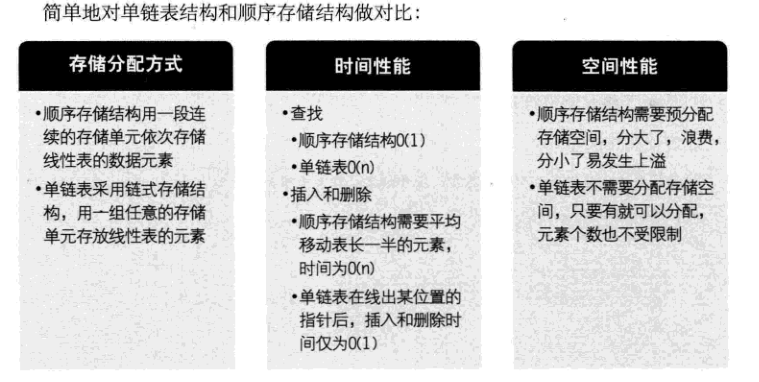• 若线性表频繁查找，很少插入和删除数据 —> 使用顺序表，反之用链表。
• 当线性表的元素个数比较大时，或者根本不知道有多大时，最好用链表。
• 如果事先知道线性表的大概长度，使用顺序表